# Algorithmic worlds

## -

Back to the information page

Introduction
Algorithmic art
Pictorial algorithms
Ultra Fractal
Algorithmic worlds
Piling patterns
The structure
Pattern generators
Index operators
The piling operator
An example
Other modules

## Long range

The principle of "Long range" requires introducing some preliminary notions. Recall that by a "pattern", I mean a real-valued function on the plane. Such an abstract pattern can be converted into a visual pattern by mean of an "index" converting each real number into a color. For pattern piling, we are mostly interested in patterns which are "quasi-periodic", in the sense that they display the same type structures everywhere on the plane. By a "shape", I will mean a pattern which is bounded: the corresponding function is simply zero outside a bounded region of the plane. It is very easy to construct such shapes. Take for instance the function which is zero outside a disk of radius R and equal to R - r at a radius r of the center of the disk. Using an index to turn into an image, we get this: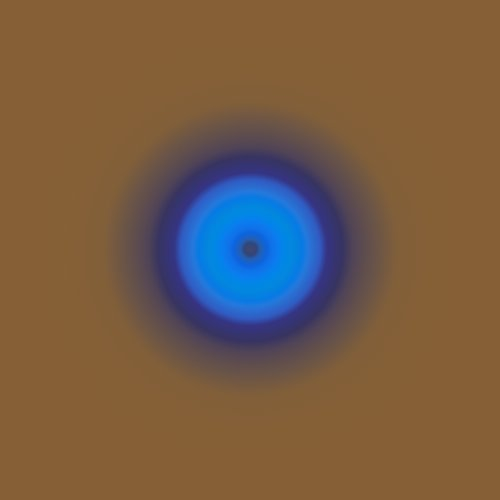A simple example of a shape. Because the points at a given distance to the center of the disk share the same value of the index, concentric rings of colors appear on the picture.

In contrast, it is difficult to draw quasi-periodic pattern which are not boringly periodic. The "Long range" pattern uses shapes to produce such quasi-periodic pattern. It proceeds as follow. Take a square gird. At each vertice, draw a shape. The last sentence really means take the function which is the sum of the functions corresponding to each shapes. This principle is not really new, it is the basis of many patterns I have been using, like for instance Invaders. What is new in the "Long range" pattern is that shapes are allowed to be larger than the mesh of the gird, what creates nice interferences effects.

Let us take the disk shape pictured above, allow both positive disks (with value R - r) and negative ones (with value r - R), and arrange them on a gird whose mesh is half their common radius. We get something like this: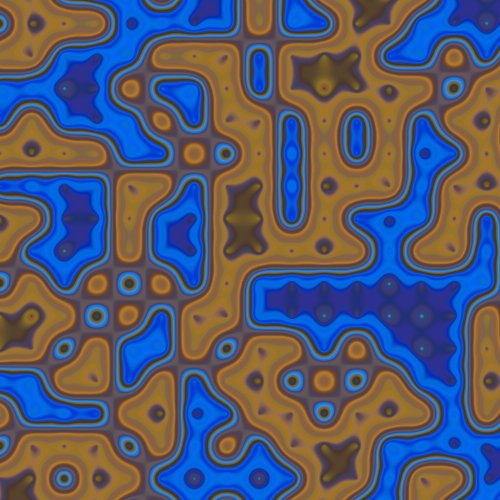An example of the Long range pattern. The shapes used are disks whose radius is twice larger than the mesh of the gird.

Somewhat interestingly, when taking the radius of the disks to be large compared to the mesh of the gird, we get patterns very reminiscent of the Perlin noise function, which is constructed very differently.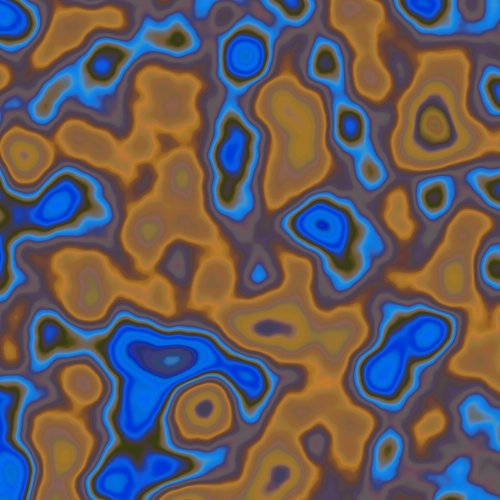An example of the Long range pattern, for which the radii of the disks are much larger than the mesh of the gird.

Of course, there is a huge number of parameters to be twisted in this algorithm. You can for instance change the shapes, weight them randomly or replace randomly some of them by zero (no shape). Here are a few interesting patterns obtained in this way.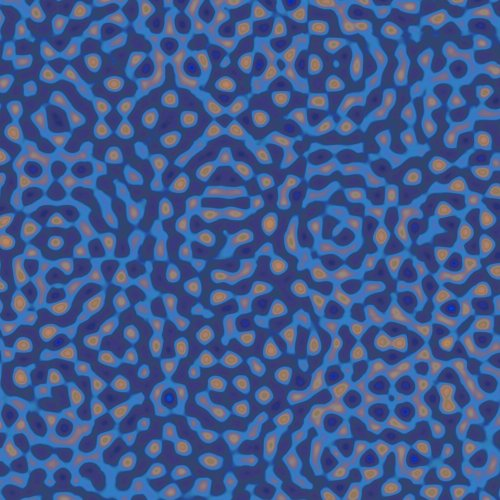The shapes used in this examples are "ripples", obtained by applying a sine function to the disk shape presented above. The shapes have been weighted randomly. This pattern is the one on which 20100926-1 is based.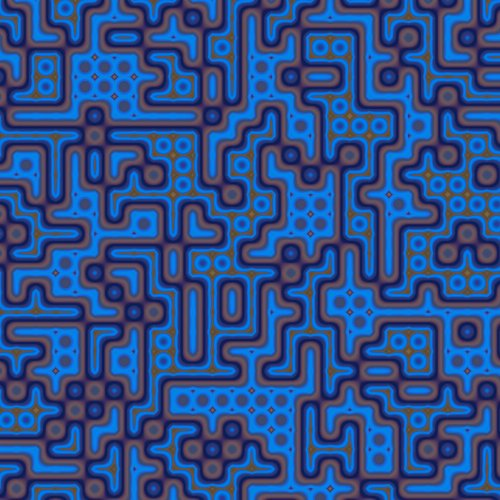Using "square ripples".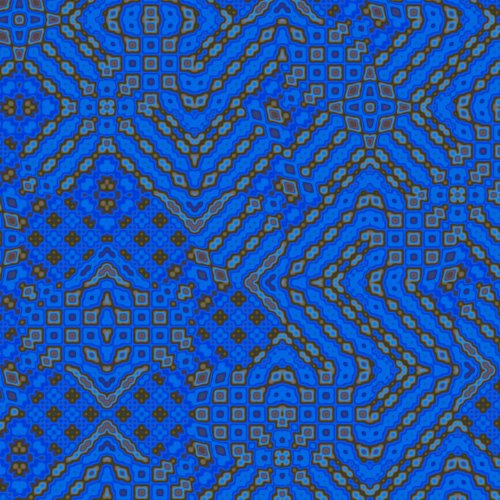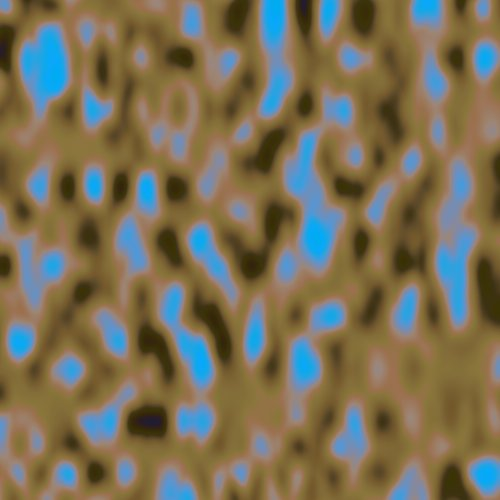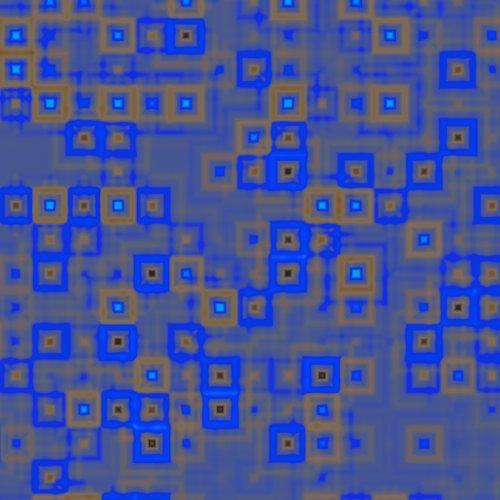Copyright S.Monnier 2009-2019. Bookmark or share